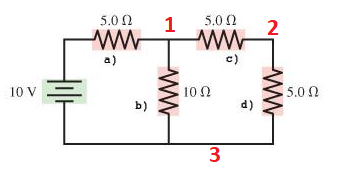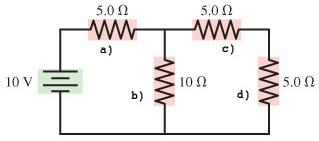# Problem: Find the current through resistor A in the figure.Find the potential difference across resistor A in the figure.Find the current through resistor B in the figure.Find the potential difference across resistor B in the figure.Find the current through resistor C in the figure.Find the potential difference across resistor C in the figure.Find the current through resistor D in the figure.Find the potential difference across resistor D in the figure.

###### FREE Expert Solution

Equivalent resistance for 2 resistors in parallel:

$\overline{){{\mathbf{R}}}_{{\mathbf{eq}}}{\mathbf{=}}\frac{{\mathbf{R}}_{\mathbf{1}}{\mathbf{R}}_{\mathbf{2}}}{{\mathbf{R}}_{\mathbf{1}}\mathbf{+}{\mathbf{R}}_{\mathbf{2}}}}$

Equivalent resistance for resistors in series:

$\overline{){{\mathbf{R}}}_{{\mathbf{eq}}}{\mathbf{=}}{{\mathbf{R}}}_{{\mathbf{1}}}{\mathbf{+}}{{\mathbf{R}}}_{{\mathbf{2}}}{\mathbf{+}}{\mathbf{.}}{\mathbf{.}}{\mathbf{.}}{\mathbf{+}}{{\mathbf{R}}}_{{\mathbf{n}}}}$

Ohm's law:

$\overline{){\mathbf{V}}{\mathbf{=}}{\mathbf{i}}{\mathbf{R}}}$Resistors c and d are in series:

Rcd = R1 + R2 = 5 + 5 = 10Ω

Resistor b is in parallel with resistors Rcd:

Rbcd = (R1R2)/(R1 + R2) = (10 × 10)/(10 + 10) = 5Ω

Resistor a is in series Rbcd:

Req = R1 + R2 = 5 + 5 = 10Ω

81% (355 ratings)###### Problem DetailsFind the current through resistor A in the figure.
Find the potential difference across resistor A in the figure.

Find the current through resistor B in the figure.
Find the potential difference across resistor B in the figure.

Find the current through resistor C in the figure.
Find the potential difference across resistor C in the figure.

Find the current through resistor D in the figure.
Find the potential difference across resistor D in the figure.